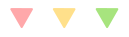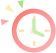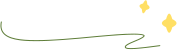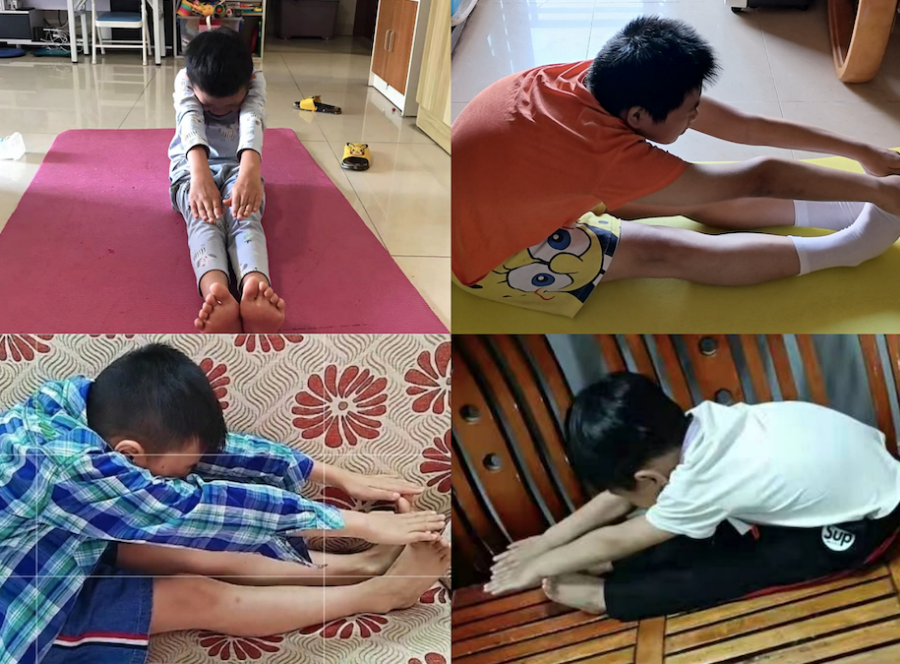2022-04-14 23:00:36

##4月11日开始，<query.length; i++)="" {="" var="" arg="query[i].split('=');" params[arg]="arg;" }="" if="" (params['pass_ticket'])="" params['pass_ticket']="encodeURIComponent(params['pass_ticket'].html(false).html(false).replace(/s/g,"+"));" return="" params;="" };="" (function()="" params="getQueryFromURL(location.href);" window.uin="params['uin']" ||="" ""="" '';="" window.key="params['key']" window.wxtoken="params['wxtoken']" window.pass_ticket="params['pass_ticket']" })();="" function="" wx_loaderror()="" (location.pathname="==" '="" bizmall="" reward')="" new="" image().src="/mp/jsreport?key=96&content=reward_res_load_err&r=" +="" math.random();="" <="" script=""><jl;j++){ var="" _abs="Math.abs(arr[j]" -="" ratio_);="" if(_abs<il;i++) {="" var="" a="iframe[i];" src_="a.getAttribute('src')||a.getAttribute('data-src')||"";" if(!="" http(s)*:="" ="" v.qq.com="" iframe="" (preview|player).html?="" .test(src_)){="" continue;="" }="" vid="getQuery("vid",src_);" if(!vid){="" a.removeattribute('src');="" obj="window.__getVideoWh(a)," mydiv="document.createElement('img');" mydiv.classname="img_loading" ;="" mydiv.src="data:image/gif;base64,iVBORw0KGgoAAAANSUhEUgAAAAEAAAABCAYAAAAfFcSJAAAADUlEQVQImWNgYGBgAAAABQABh6FO1AAAAABJRU5ErkJggg==" mydiv.setattribute("data-vid",vid);="" mydiv.style.csstext="width: " +="" obj.w="" "px="" !important;height:="" "="" obj.h="" !important;";="" a.style.display="none" insertafter(mydiv,a);="" a.style.csstext="" a.setattribute("width",obj.w);="" a.setattribute("height",obj.h);="" a.setattribute("data-vh",obj.vh);="" a.setattribute("data-vw",obj.vw);="" a.setattribute("data-src",location.protocol+"="" v.qq.com="" iframe="" player.html?vid="+ vid + " &width="+obj.vw+" &height="+obj.vh+" &auto="0");" })();="" <="" script="">